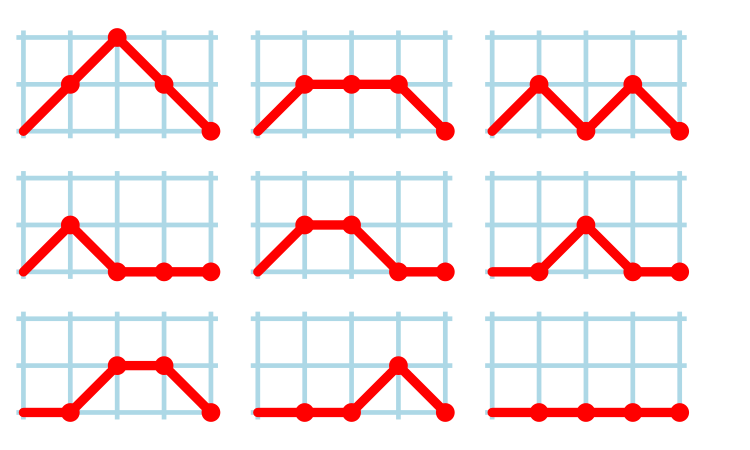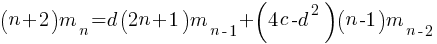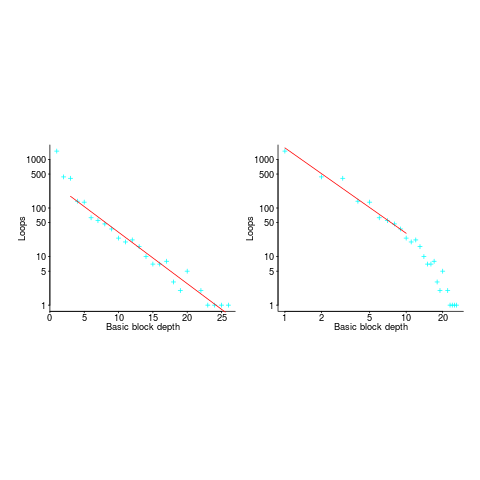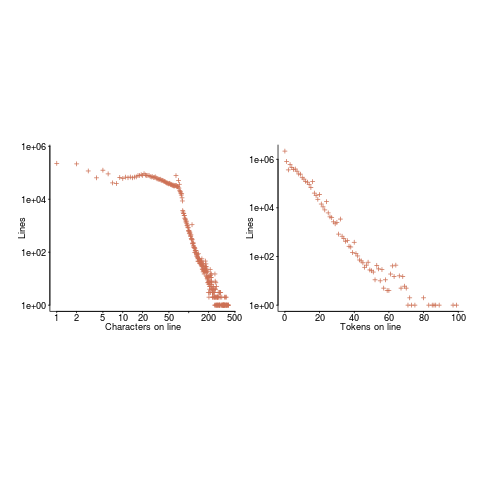## Motzkin paths and source code silhouettes

Consider a language that just contains assignments and if-statements (no `else` arm). Nesting level could be used to visualize programs written in such a language; an `if` represented by an Up step, an `assignment` by a Level step, and the if-terminator (e.g., the `}` token) by a Down step. Silhouettes for the nine possible four line programs are shown in the figure below (image courtesy of Wikipedia). I use the term silhouette because the obvious terms (e.g., path and trace) have other common usage meanings.How many silhouettes are possible, for a function containingstatements? Motzkin numbers provide the answer; the number of silhouettes for functions containing from zero to 20 statements is: 1, 1, 2, 4, 9, 21, 51, 127, 323, 835, 2188, 5798, 15511, 41835, 113634, 310572, 853467, 2356779, 6536382, 18199284, 50852019. The recurrence relation for Motzkin numbers is (whereis the total number of steps, i.e., statements):Human written code contains recurring patterns; the probability of encountering an if-statement, when reading code, is around 17% (at least for the C source of some desktop applications). What does an upward probability of 17% do to the Motzkin recurrence relation? For many years I have been keeping my eyes open for possible answers (solving the number theory involved is well above my mathematics pay grade). A few days ago I discovered weighted Motzkin paths.

A weighted Motzkin path is one where the Up, Level and Down steps each have distinct weights. The recurrence relationship for weighted Motzkin paths is expressed in terms of number of colored steps, where:is the number of possible colors for the Level steps, andis the number of possible colors for Down steps; Up steps are assumed to have a single color:setting:and(i.e., all kinds of step have one color) recovers the original relation.

The different colored Level steps might be interpreted as different kinds of non-nesting statement sequences, and the different colored Down steps might be interpreted as different ways of decreasing nesting by one (e.g., a `goto` statement).

The connection between weighted Motzkin paths and probability is that the colors can be treated as weights that add up to 1. Searching on “weighted Motzkin” returns the kind of information I had been looking for; it seems that researchers in other fields had already discovered weighted Motzkin paths, and produced some interesting results.

If an automatic source code generator outputs the start of an `if` statement (i.e., an Up step) with probability, an assignment (i.e., a Level step) with probability, and terminates the `if` (i.e., a Down step) with probability, what is the probability that the function will contain at leaststatements? The answer, courtesy of applying Motzkin paths in research into clone cell distributions, is:where:is the‘th Catalan number, and that `[...]` is a truncation; code for an implementation in R.

In human written code we know that, because the number of statements in a compound-statement roughly has an exponential distribution (at least in C).

There has been some work looking at the number of peaks in a Motzkin path, with one formula for the total number of peaks in all Motzkin paths of length n. A formula for the number of paths of length, havingpeaks, would be interesting.

Motzkin numbers have been extended to what is called higher-rank, where Up steps and Level steps can be greater than one. There are statements that can reduce nesting level by more than one, e.g., `break`ing out of loops, but no constructs increase nesting by more than one (that I can think of). Perhaps the rather complicated relationship can be adapted to greater Down steps.

Other kinds of statements can increase nesting level, e.g., for-statements and while-statements. I have not yet spotted any papers dealing with the case where an Up step eventually has a corresponding Down step at the appropriate nesting level (needed to handle different kinds of nest-creating constructs). Pointers welcome. A related problem is handling if-statements containing `else` arms, here there is an associated increase in nesting.

What characteristics does human written code have that results in it having particular kinds of silhouettes? I have been thinking about this for a while, but have no good answers.

If you spot any Motzkin related papers that you think could be applied to source code analysis, please let me know.

## for-loop usage at different nesting levels

When reading code, starting at the first line of a function/method, the probability of the next statement read being a `for-loop` is around 1.5% (at least in C, I don’t have decent data on other languages). Let’s say you have been reading the code a line at a time, and you are now reading lines nested within various `if`/`while`/`for` statements, you are at nesting depth. What is the probability of the statement on the next line being a `for-loop`?

Does the probability of encountering a `for-loop` remain unchanged with nesting depth (i.e., developer habits are not affected by nesting depth), or does it decrease (aren’t developers supposed to using functions/methods rather than nesting; I have never heard anybody suggest that it increases)?

If you think the `for-loop` use probability is not affected by nesting depth, you are going to argue for the plot on the left (below, showing number of loops appearing in C source at various nesting depths), with the regression model fitting really well after 3-levels of nesting. If you think the probability decreases with nesting depth, you are likely to argue for the plot on the right, with the model fitting really well down to around 10-levels of nesting (code+data).Both plots use the same data, but different scales are used for the x-axis.

If probability of use is independent of nesting depth, an exponential equation should fit the data (i.e., the left plot), decreasing probability is supported by a power-law (i.e, the right plot; plus other forms of equation, but let’s keep things simple).

The two cases are very wrong over different ranges of the data. What is your explanation for reality failing to follow your beliefs in `for-loop` occurrence probability?

Is the mismatch between belief and reality caused by the small size of the data set (a few million lines were measured, which was once considered to be a lot), or perhaps your beliefs are based on other languages which will behave as claimed (appropriate measurements on other languages most welcome).

The nesting depth dependent use probability plot shows a sudden change in the rate of decrease in `for-loop` probability; perhaps this is caused by the maximum number of characters that can appear on a typical editor line (within a window). The left plot (below) shows the number of lines (of C source) containing a given number of characters; the right plot counts tokens per line and the length effect is much less pronounced (perhaps developers use shorter identifiers in nested code). Note: different scales used for the x-axis (code+data).I don’t have any believable ideas for why the exponential fit only works if the first few nesting depths are ignored. What could be so special about early nesting depths?

What about fitting the data with other equations?

A bi-exponential springs to mind, with one exponential driven by application requirements and the other by algorithm selection; but reality is not on-board with this idea.

Ideas, suggestions, and data for other languages, most welcome.